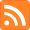# The Tiger's Stripes

A technical blog on math, computer science, and game theory.

Author: Bo Waggoner RSS feed# Subgaussian Variables and Concentration

Posted: 2017-12-18.

In this post we'll take a look at the definition of subgaussian random variables and see how those are used in measure concentration.

We'll see a couple advantages of the definitions and studying subgaussians:

• The point is that subgaussian variables have "tails" that fall off like Gaussians with exponentially decreasing probability, depending on their subgaussian parameter.
• Basic operations, like scaling by a constant or adding together independent variables, give new subgaussian parameters according to simple formulas.
• The subgaussian tail bounds for these variables are generally very tight, just like Chernoff or Hoeffding bounds.

## Basics and Definition

First, you should be familiar with the basics of measure concentration. We have a random variable $Y$ taking values in $\mathbb{R}$. We will assume here that $Y$ has mean zero although one can naturally extend the definitions by subtracting off the mean.

If $Y$ has mean zero, $Y$ is said to be $\sigma^2$-subgaussian if for all $b \in \mathbb{R}$,

$\mathbb{E}[e^{bY}] \leq e^{\sigma^2 b^2/2} .$

Fact 1. If $Y$ is $\sigma^2$-subgaussian, then for all $t \geq 0$, $\Pr[ Y \geq t ] \leq e^{-t^2 / (2\sigma^2)}$ and $\Pr[ Y \leq -t ] \leq e^{-t^2 / (2\sigma^2)} .$

Proof. By Markov's inequality and the Chernoff method'', for all $\theta \gt 0$, \begin{align*} \Pr[ Y \geq t ] &= \Pr[ e^{\theta Y} \geq e^{\theta t} ] \\ &\leq e^{-\theta t} \mathbb{E} e^{\theta Y} \\ &\leq e^{-\theta t} e^{\sigma^2 \theta^2 / 2} \\ &= e^{\frac{1}{2}\sigma^2 \theta^2 - \theta t} \\ &= e^{- t^2 / (2\sigma^2)} . \end{align*} by choosing $\theta = t/\sigma^2$. The proof of the second inequality is analogous.

## The Caclulus of Subgaussians

Subgaussians satisfy nice additivity and scaling properties:

Fact 2. Let $Y_1, Y_2$ be mean-zero and independent, and respectively $\sigma_1^2$, $\sigma_2^2$-subgaussian. Then

1. $\alpha Y_1$ is $\alpha^2 \sigma_1^2$-subgaussian.
2. $Y_1 + Y_2$ is $\sigma_1^2 + \sigma_2^2$-subgaussian.

Proof.

(1) Fixing $\alpha$:

\begin{align*} \mathbb{E} e^{b (\alpha Y_1)} &= \mathbb{E} e^{(b\alpha) Y_1} \\ &\leq e^{(b^2 \alpha^2) \sigma^2 / 2} \\ &= e^{b^2 (\alpha^2 \sigma^2) / 2} . \end{align*}

(2) By independence:

\begin{align*} \mathbb{E} e^{b(Y_1 + Y_2)} &= \mathbb{E} e^{bY_1} \mathbb{E} e^{bY_2} \\ &\leq e^{b^2 \sigma_1^2/2} e^{b^2\sigma_2^2/2} \\ &= e^{b^2 (\sigma_1^2 + \sigma_2^2)/2}. \end{align*}

In particular, for example, the average of $n$ independent variables each $\sigma^2$-subgaussian will be $(\sigma^2/n)$-subgaussian.

## Examples

Example 1: If $|Y| \leq 1$ with probability $1$ and has mean zero, then $Y$ is $1$-subgaussian.

Proof.

This is actually a special case of Hoeffding's Lemma and we follow a standard proof. By convexity, for each $y \in [-1,1]$ we have for $\alpha = \frac{1 + y}{2}$, \begin{align*} e^{by} &= e^{\alpha(b) + (1-\alpha)(-b)} \\ &\leq \alpha e^b + (1-\alpha)e^{-b} \\ &= \frac{1+y}{2} e^b + \frac{1-y}{2} e^{-b} \end{align*} so \begin{align*} \mathbb{E} e^{by} &\leq \frac{1+\mathbb{E}y}{2} e^b + \frac{1-\mathbb{E}y}{2} e^{-b} \\ &= \frac{1}{2}e^b + \frac{1}{2}e^{-b} \\ &= \text{cosh}(b) \\ &\leq e^{-b^2/2} \end{align*} by the `cosh'' inequality.

Note that combined with Fact 2, this gives that any mean-zero variable lying within $[-a,a]$ is $a^2$-subgaussian.

These facts alone can recover some powerful and popular tail bounds such as Hoeffding's bound. Namely, if each $X_1,\dots,X_n$ is in $[-1,1]$ and has mean $0$, and they are all independent, then their sum is $n$-subgaussian and their average is $(1/n)$-subgaussian, so by Fact 1, the average differs from its expectation by $t$ with probability only $e^{-nt^2/2}$.

Example 2: If $Y$ is Gaussian$(0,\sigma^2)$, then $Y$ is $\sigma^2$-subgaussian.

Proof. Recall the density of $Y$ is $f(y) = \alpha e^{-y^2/(2\sigma^2)}$ where $\alpha = \frac{1}{\sigma\sqrt{2\pi}}$. So \begin{align*} \mathbb{E} e^{bY} &= \alpha \int_{y=-\infty}^{\infty} e^{by} e^{-y^2/(2\sigma^2)} dy \\ &= \alpha \int \exp\left[ -\frac{1}{2} \left( \frac{y^2}{\sigma^2} - 2by + b^2\sigma^2 - b^2\sigma^2 \right) \right] dy \\ &= \alpha \int \exp\left[ -\frac{1}{2} \left( \frac{y}{\sigma} - b\sigma\right)^2 + \frac{b^2\sigma^2}{2} \right] dy \\ &= e^{b^2\sigma^2/2} \alpha \int e^{ -\frac{1}{2\sigma^2}\left( y - b\sigma^2\right)^2 } dy \\ &= e^{b^2\sigma^2/2} . \end{align*} At the end, we used that the integral, if we include the factor $\alpha$, is over the probability density function of a Gaussian with mean $b\sigma^2$ and variance $\sigma^2$, so it evaluates to $1$.

Note in the last proof, every step held with equality.

Limits to 1000 characters. HTML does not work but LaTeX does, e.g. $\$$x^y\$$ displays as$x^y\$.